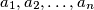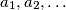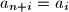### IMO Shortlist 2013 problem A4

Kvaliteta:
Avg: 0,0
Težina:
Avg: 7,0
Let$n$ be a positive integer, and consider a sequence$a_1, a_2, \ldots, a_n$ of positive integers. Extend it periodically to an infinite sequence$a_1, a_2, \ldots$ by defining$a_{n+i} = a_i$ for all$i \geq 1$. Ifandprove thatIzvor: Germany